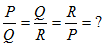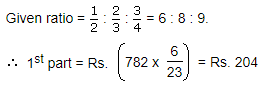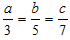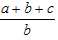Courses

# Ratio And Proportion MCQ

## 30 Questions MCQ Test Quantitative Techniques for CLAT | Ratio And Proportion MCQ

Description
This mock test of Ratio And Proportion MCQ for Quant helps you for every Quant entrance exam. This contains 30 Multiple Choice Questions for Quant Ratio And Proportion MCQ (mcq) to study with solutions a complete question bank. The solved questions answers in this Ratio And Proportion MCQ quiz give you a good mix of easy questions and tough questions. Quant students definitely take this Ratio And Proportion MCQ exercise for a better result in the exam. You can find other Ratio And Proportion MCQ extra questions, long questions & short questions for Quant on EduRev as well by searching above.
QUESTION: 1

### If P : Q : = 8 : 15 and Q : R = 3 : 2, then find P : Q : R

Solution:

Given that, P : Q = 8 : 15, Q : R = 3 : 2

P : Q : R = (8 x 3) : (15 x 3) : (15 x 2) = 24 : 45 : 30

∴ P : Q : R = 8 : 15 : 10

Here, consequent of first ratio should be equal to the antecedent of second ratio.

QUESTION: 2

Solution:
QUESTION: 3

### Find the 4th proportional to 4, 16 and 7

Solution:
QUESTION: 4

Find the 3rd proportion to 15 and 30

Solution:
QUESTION: 5

Find the mean proportional between 9 and 64.

Solution:
QUESTION: 6

Find the compound ratio of 2:7, 5:3, 4:7

Solution:
QUESTION: 7

A : B is 3 : 4 and B : C  is 8 : 9 Find A : B : C

Solution:
QUESTION: 8

If 4a = 5 b and 7b = 9c then a : b : c = ?

Solution:
QUESTION: 9

If P : Q : R = 2 : 3 : 4  thenSolution:
QUESTION: 10

a : b = c : d = e : f = 1 : 2 then (3a + 5c + 7e) : (3b + 5d + 7f) = ?

Solution:
QUESTION: 11

The average age of a person and his son is 30 years. The ratio of their age is 4 : 1 respectively. Find the age of son.

Solution:
QUESTION: 12

If Rs. 782 be divided into three parts, proportional to 1/2 = 2/3 = 3/4  then the first part is:

Solution:QUESTION: 13

A bag contains one rupee, 50 paise 25 paisa coins in the Ratio of 8:9:11. If the total money in the bag is Rs. 366, find the number of 25 paisa coins.

Solution:

Let coins are
1 ruppe coin = 8X.
Value of 1 ruppe coin = 8X.
50 paise coins = 9X
Value of 50 paise coin = 9X/2
25 paise coins = 11X
Value of 25 paise coins = 11X/4.
Total Ruppes = 366
8X + 9X/2 + 11X/4 = 366
61X/4 = 366
61X = 1464
X = 24.
Number of 25p paise coin = 11X = 11* 24 = 264.

QUESTION: 14

A mixture contains alcohol and water in the ratio of 4 : 3. If 14 L of water is added to the mixture the ratio of Alcohol and water becomes 3 : 4, find the quantity of alcohol in the mixture.

Solution:
QUESTION: 15

Rahul obtained 12 marks more than that of Tanvi. If the ratio of their marks is 4:3. Find the sum of their marks.

Solution:
QUESTION: 16

One half of a certain number is equal to 65% of the second number. Find the ratio of Ist number to 2nd number.

Solution:
QUESTION: 17

If, then find the value ofSolution:
QUESTION: 18

Monthly income of P and Q are in the ratio 2 : 3 and their monthly expenses are in the ratio of 5 : 9. If each of them saves Rs 300 per month, find their respective monthly income

Solution:
QUESTION: 19

What number has to be added to each term of 3 : 5 to make the ratio 5 : 6?

Solution:
QUESTION: 20

The ratio between two numbers is 3 : 4. If each number is increased by 3, the ratio becomes 4 : 5. Find the difference between the numbers.

Solution:
QUESTION: 21

In a class, the number of boys and girls is in the ratio of 4 : 5. If 10 more boys join the class, the ratio of boys to girls becomes 6 : 5. How many girls are there in the class.

Solution:
QUESTION: 22

The sum of the ages of a father and his son is 100 yrs now. Five years ago their ages were in the ratio 2 : 1. Find the ratio of ages of father and son after 10 years.

Solution:
QUESTION: 23

The average age of a woman and her daughter is 19 years. The ratio of their ages is 16 : 3. What is the age of the daughter?

Solution:

Woman’s age = 16x years
Her daughter’s age = 3x years
∴ 16x + 3x = 2 × 19 = 38
⇒ 19x = 38 ⇒ x = 2
∴  Daughter’s age = 3 × 2

QUESTION: 24

The ages of A and B are in the ratio 12 : 7. After 6 years the ratio will become 3 : 2. What is the difference in their ages

Solution:
QUESTION: 25

The average age of a man and his two sons, born on the same day, is 30 yr. The ratio of the ages of father to one of the sons is 5 : 2. What is the father’s age.

Solution:
QUESTION: 26

The ratio of present ages of Seeta and Geeta is 8 : 9. The sum of their ages is 68. What will be the ratio of their ages after 10 years?

Solution:
QUESTION: 27

The ratio between the present ages of Punit and suresh is 4 : 5. After 7 years Punit’s age will be 31 years. What was Suresh’s age four years ago

Solution:
QUESTION: 28

In a town 80% of the population are adults of which men and women are in the ratio of 9 : 7 respectively. If the number of adult women is 4.2 Lakh, what is the total population of the town.

Solution: Let the number of adult men be N.
9 : 7 = N : 4.2
⇒ N = (9/7) x 4.2 = 5.4 Lakh
Total adult population = 4.2 + 5.4 = 9.6 lakh.

If the population of the town be M Lakh,
then, 80M/100 = 9.6
⇒ M = (9.6 x 100)/80 = 12 lakh
QUESTION: 29

If the positions of the digits of a two digit no. are interchanged the number newly formed is smaller than the original number by 45. Also the ratio of new number to original number is 3 : 8. What is the original number

Solution:
QUESTION: 30

There are two triangles A and B. The angles of triangle A are in the ratio 3 : 4 : 5 and the angles of triangle B are in the ratio of 5 : 6 : 7. What is the difference between the largest angle of triangle A and the smallest angle of triangle B.

Solution: The sum of all angles of triangle is 180 degrees .
so , in triangle A , let the sides be 3x,4x,5x
3x+4x+5x=180
12x=180 therefore x=15.
so, the angles are 3x = 45, 4x=60, 5x=75
Similarly in triangle B
5x+6x+7x=180
so x=10
angles are 50,60,70
largest angle of triangle A is 75 and smallest of B is 50 and the difference is 25 .